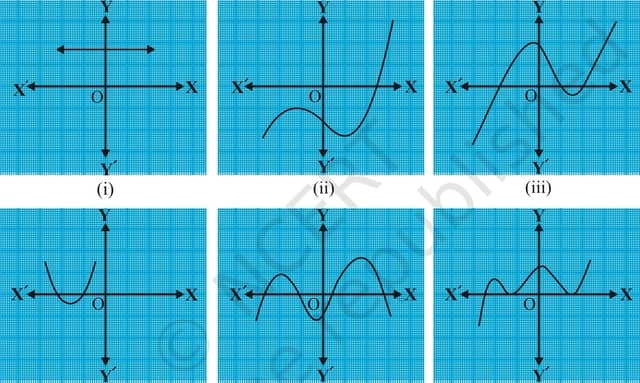Most of the important things in the world have been accomplished by people who have kept on trying when there seemed to be no help at all. – Dale Carnegie

Meaning of Polynomials (Linear, quadratic and cubic polynomials)

Graphical representation of different types of quadratic polynomials and how to read number of zeroes from graph of quadratic equation

00:07:04 NCERT Exercise 2.1 Question 100:08:45 Explanation for Relationship between coefficients and zeroes (roots) of a quadratic polynomial

00:10:45 Derivation for relationship between coefficients and roots of quadratic polynomial

NCERT Exercise 2.2 Question 1 Find the zeroes of the following quadratic polynomials and verify the relationship between the zeroes and the coefficients.
(i) x^2-2x-8
(ii) 4s^2-4s+1
(iii) 6x^2-3-7x
(iv) 4u^2+8u
(v) t^2-15
(vi) 3x^2-x-4